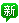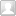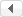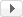用户名 Email 密码 记住 立即注册找回密码

##搜索

# [说明书] 重组胰蛋白酶RPT0201 的介绍发表于 2021-12-14 16:10 | 显示全部楼层 |阅读模式
x

Cat.No.：RPT0201
CAS9002-07-7
EC3.4.21.4

1.产品简介

2.产品特性
 [size=10.5000pt]来源[size=10.5000pt] [size=10.5000pt]重组大肠杆菌[size=10.5000pt] [size=10.5000pt]产品[size=10.5000pt]外观[size=10.5000pt] [size=10.5000pt]白色、类白色、类黄色粉末[size=10.5000pt] [size=10.5000pt]比活[size=10.5000pt] [size=10.5000pt]≥[size=10.5000pt]3800 [size=10.5000pt]USP[size=10.5000pt] [size=10.5000pt]u[size=10.5000pt]nits[size=10.5000pt]/mg pro[size=10.5000pt].[size=10.5000pt] [size=10.5000pt]纯度（蛋白电泳）[size=10.5000pt] [size=10.5000pt]单一主条带[size=10.5000pt] [size=10.5000pt]分子量（蛋白电泳）[size=10.5000pt] [size=10.5000pt]24[size=10.5000pt].[size=10.5000pt]0[size=10.5000pt]±2.4[size=10.5000pt] [size=10.5000pt]kDa[size=10.5000pt]

3.使用方法
1mMHCl溶解，重组胰蛋白酶浓度为1-10mg/ml 。使用时，酶量：目的蛋白=150-11000，最适pH7.0-11.0
4.储存和运输稳定性

5.产品优势

6.产品用途

## 发表回复

 本版积分规则 回帖并转播 回帖后跳转到最后一页

## 官方推荐/3